# Energy-Mass Equivalence

This is part of the HSC Physics course under the topic Light and Special Relativity.

### HSC Physics Syllabus

• Use Einstein’s mass–energy equivalence relationship E=mc^2 to calculate the energy released by processes in which mass is converted to energy, for example: (ACSPH134)
– production of energy by the sun
– particle–antiparticle interactions, eg positron–electron annihilation
– combustion of conventional fuel

### Energy and Mass Equivalence

In classical physics, the law of energy and mass conservation underpins many common phenomena such as the collision between objects, acceleration of rockets during its launch and all chemical reactions (formation of product from reactants)

In addition to the special theory of relativity, Einstein also proposed that the two quantities (mass and energy) are in fact interchangeable. Mass can be converted into energy and energy can be converted into mass. As a result, each quantity does not need to be conserved in its own form as they can inter-convert.

The energy and mass equivalence is governed by the equation:

$$E=mc^2$$

• Where E is energy in Joules (J)
• m is the rest mass of a particle or object in kilograms (kg)
• c is the speed of light in m s-1

Alternatively, this equation can be used by using the following units:

• E in MeV
• in MeV c^(-2)

Mass can be converted from kg to atomic mass unit (u) by dividing by 1.661 xx 10^(-27).

It can be converted from u to MeV c^(-2) by multiplying by 931.5.

This form of mass and energy conservation and conversion is commonly observed in nuclear reactions and situations where high-speed particles collide.

### Production of energy by the Sun

Stars, including our Sun, produce energy via nuclear fusion. Nuclear fusion is the process in which atomic nuclei physically combine to form one or more different atomic nucleus and additional sub-atomic particles such as neutrons.

Nuclear fusion obeys energy-mass conservation but requires the application of energy-mass equivalence.

Proton-proton Chain

The simplest form of nuclear fusion occurs between hydrogen nuclei to form helium. The atomic mass of one hydrogen atom is roughly 1.008 u. When four hydrogen atoms combine, the total mass of resultant products should add up to 4.032 u due to mass conservation.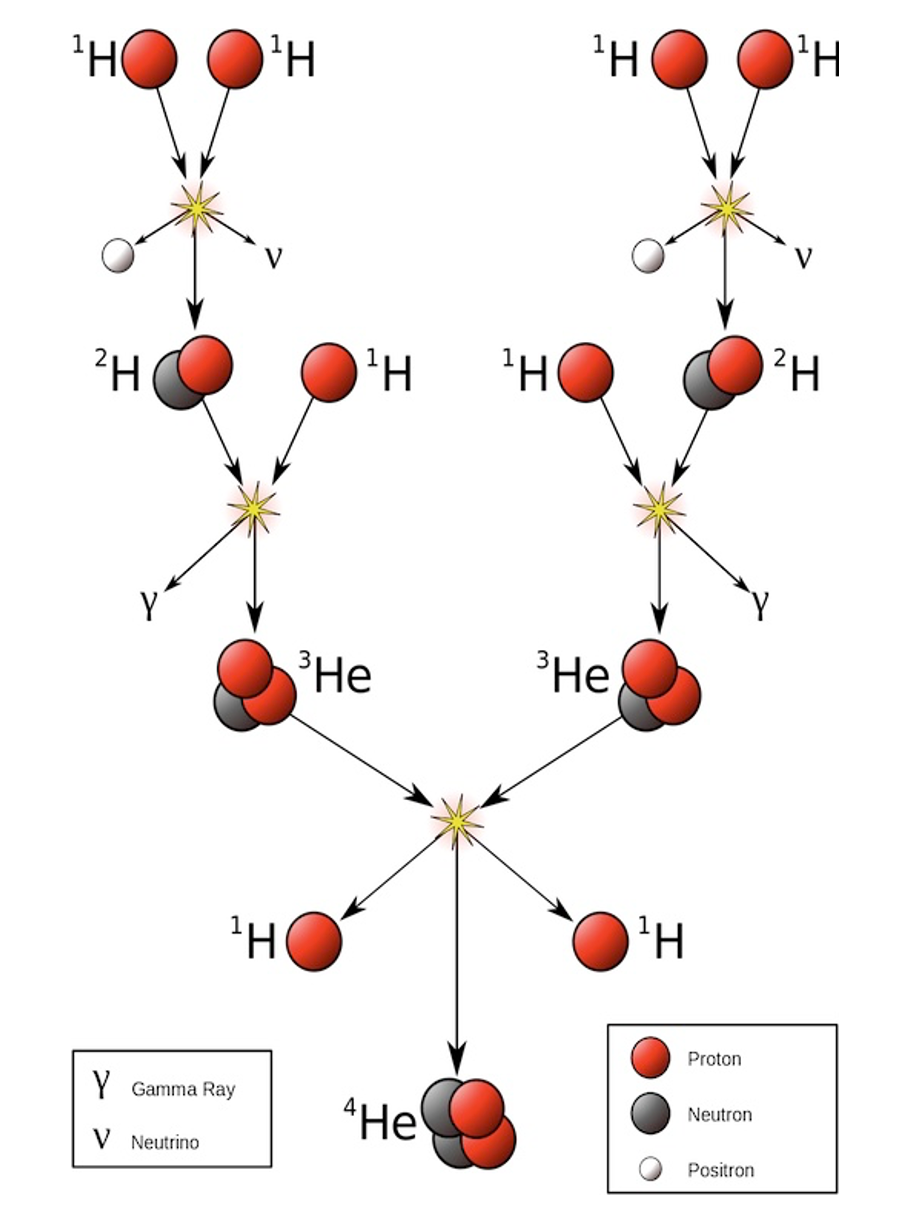However, the resultant helium nucleus weighs less than 4.032 u which means the lost mass has converted into energy. Using Einstein’s equation and information from the periodic table, the energy produced isThe overall reaction of the proton-proton chain can be summarised by the following nuclear equation.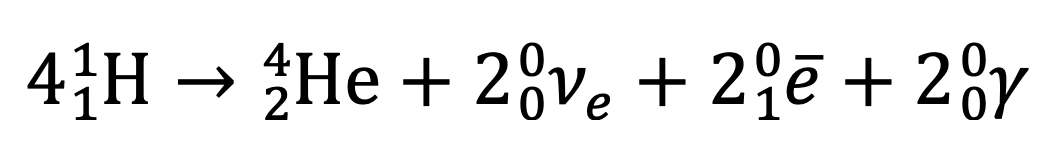Four protons are combined to form a He-4 nucleus, two electron neutrinos, two positrons and two gamma photons.

Carbon, Nitrogen, Oxygen (CNO) Cycle

CNO cycle is a series of nuclear fusion reactions that occur in main sequence stars like our Sun. The cycle involves isotopes of carbon, nitrogen and oxygen, and more importantly the production of a helium nucleus from the inclusion of four protons in different parts of the cycle.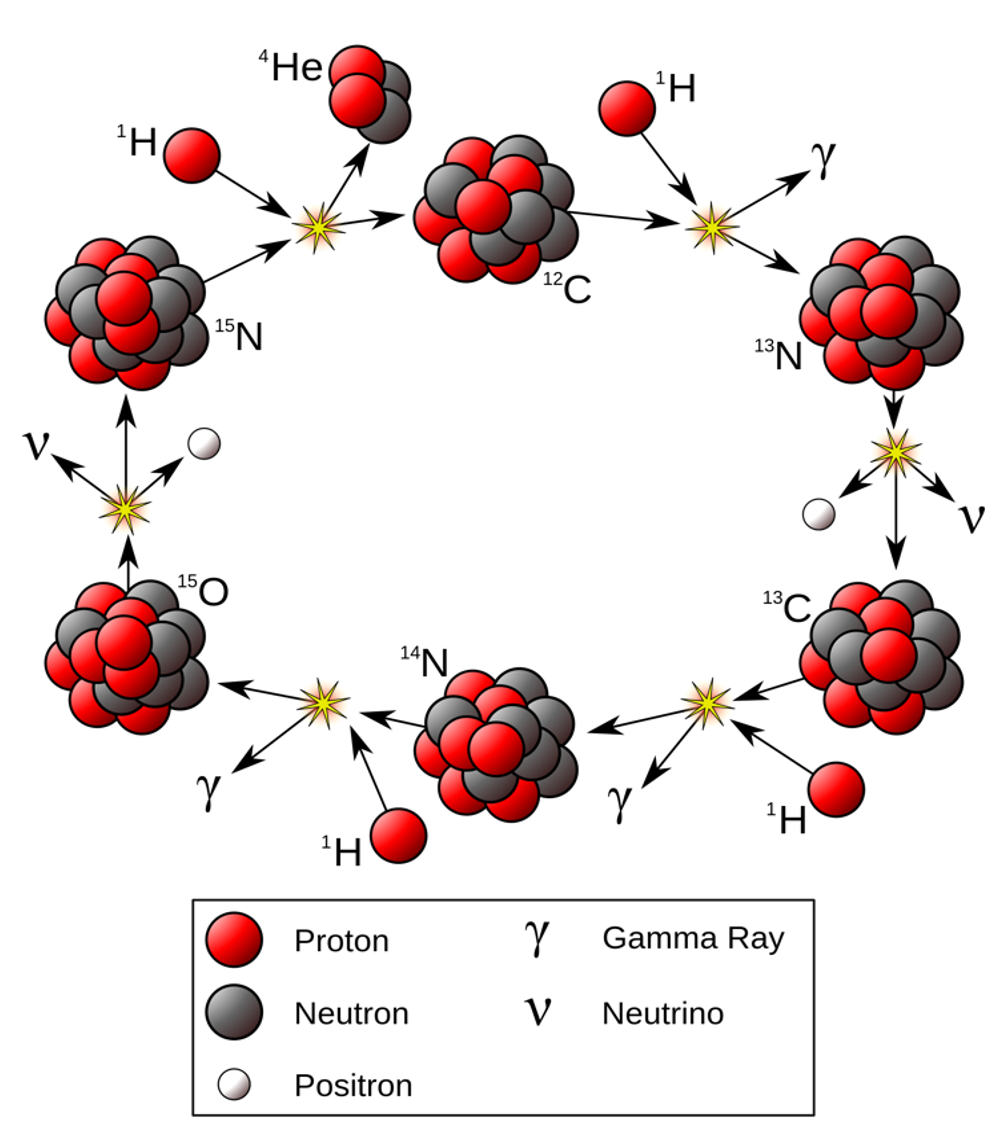The application of energy-mass equivalence in CNO cycle is identical to that in the proton-proton chain. The energy is derived from the mass difference between four protons and the result product of nuclear fusion – helium nucleus.

### Particle-antiparticle Annihilation

Annihilation is a process in which a particle collides with its anti-particle to produce two identical photons. A particle and its antiparticle have the same mass but opposite charge.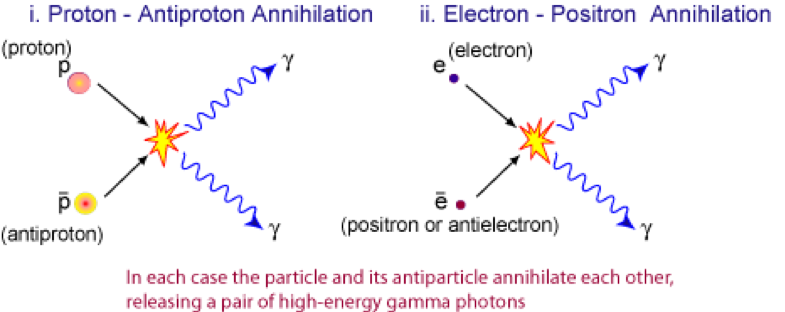Electron-positron annihilation

Collision between an electron and positron produces two photons with frequencies equivalent to those of gamma radiation.

The rest energy of an electron and positron are equal because they possess equal mass.

The energy of an electron/positron is given by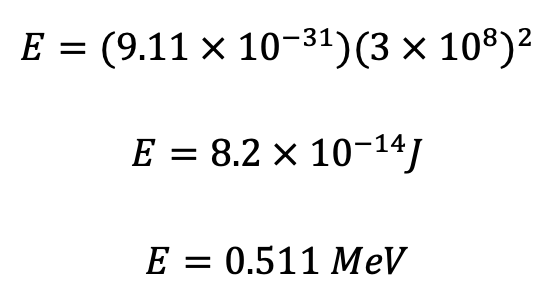The photons produced (gamma rays) each possess the same amount of energy of 0.511 MeV. It is important to note that Planck’s quantum theory (E = hf) can be used calculate the frequency and wavelength associated with a photon of this energy level.

The total energy (two photons combined) is the sum of energy transformed from the mass of electron and positron.

$$E_{total} = 0.511 \times 2 = 1.022 \hspace{0.1cm} MeV$$

Proton-antiproton annihilation

The collision between a proton and antiproton is more complicated than the annihilation between electron and positron. This is because a proton is a baryon consisting of multiple (three to be exact) quarks whereas an electron has a simpler structure.

Due to protons and antiprotons’ more complex structure, the collision involves several interactions and thus, produces more than just photons as energy. The annihilation can also produce electrons, positrons and neutrinos.

The total energy produced can be calculated using the combined mass of a proton and an antiproton:

$$E_{total}=(2 \times 1.673 \times 10^{-27})(3 \times 10^8)^2$$

$$E_{total}=3.01 \times 10^{-10} \hspace{0.1cm} J$$

$$E_{total}=1880 \hspace{0.1cm} MeV$$

### Combustion of Conventional Fuel

Combustion of fuel is a common application of transforming chemical energy into thermal energy and/or kinetic energy. The difference in mass between products and reactants can be accounted for by considering the energy produced.

Octane is a common fuel found at petrol stations. Its combustion reaction is outlined below.The mass of products has been observed to be smaller than the amount of fuel and oxygen gas consumed. However, the mass difference is much harder to determine compared to nuclear reactions as combustion reactions occur on a macroscopic scale.

Previous section: Relativistic Momentum